Integration by partial fraction - Type 1

Chapter 7 Class 12 Integrals
Concept wise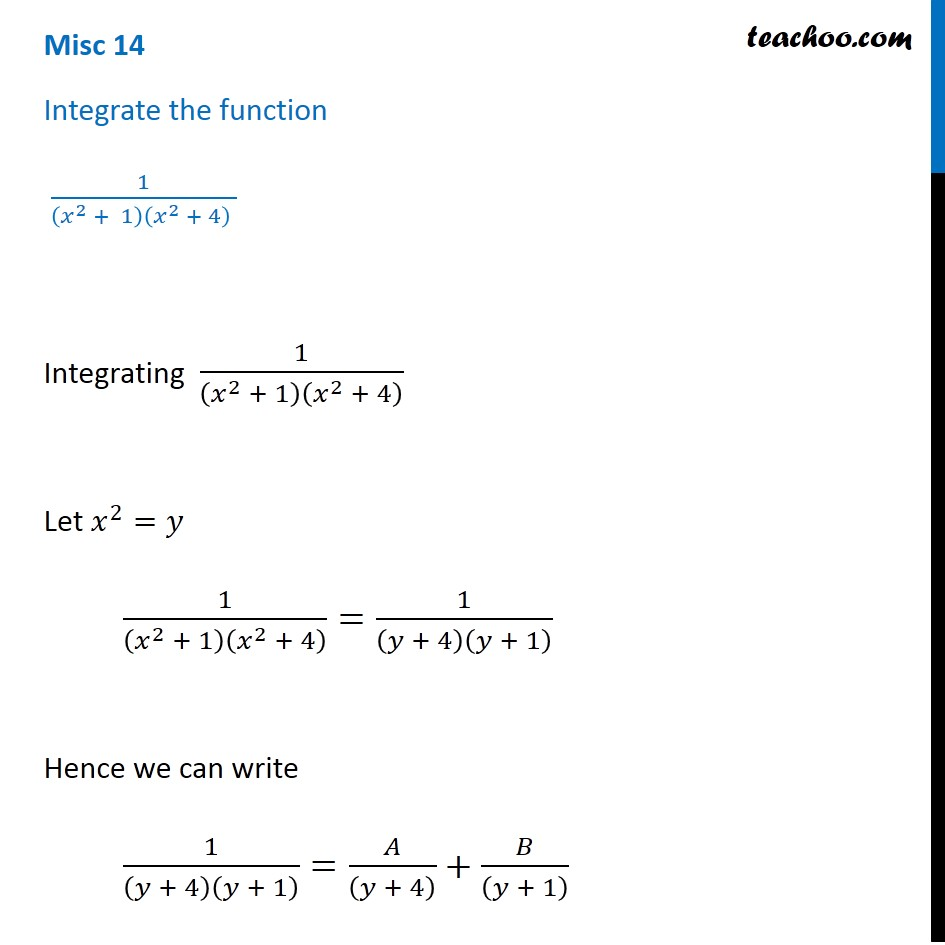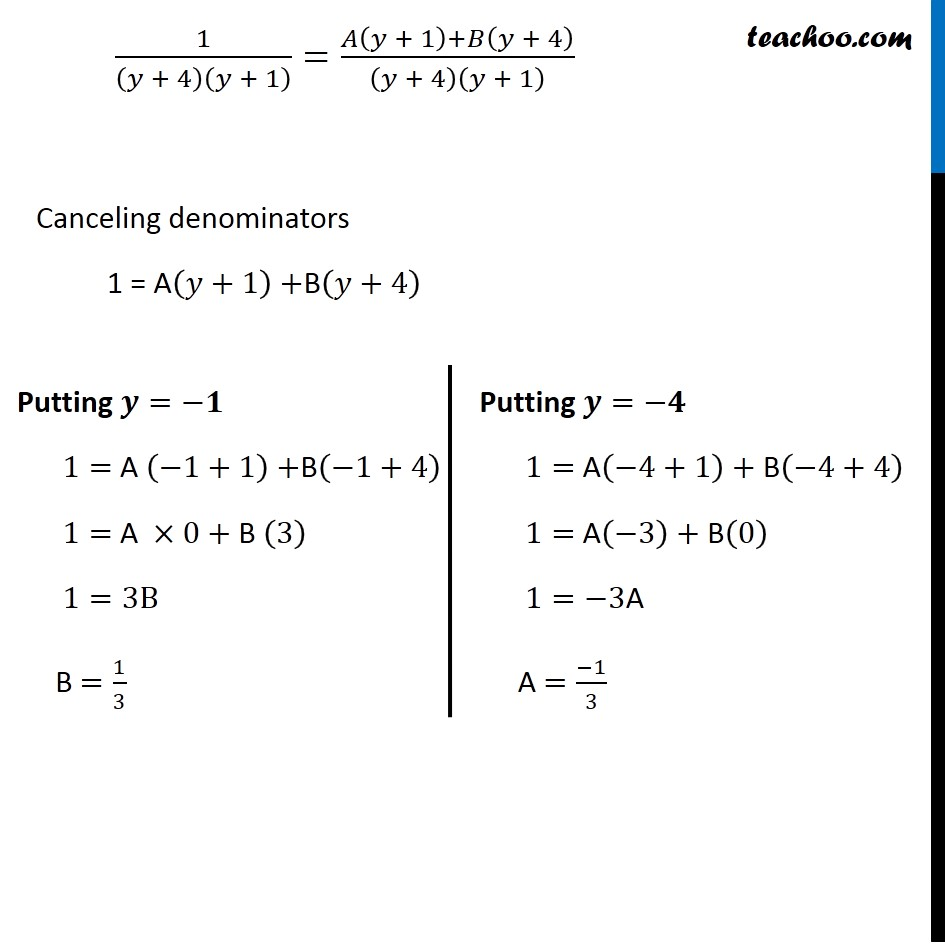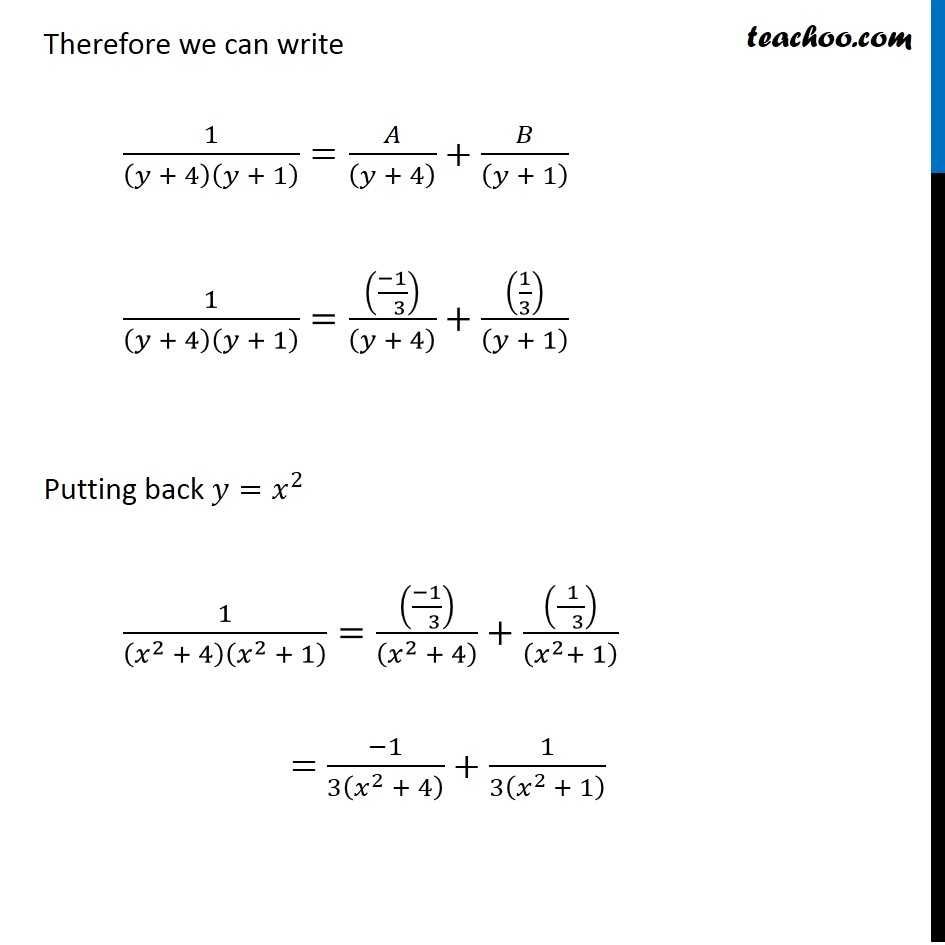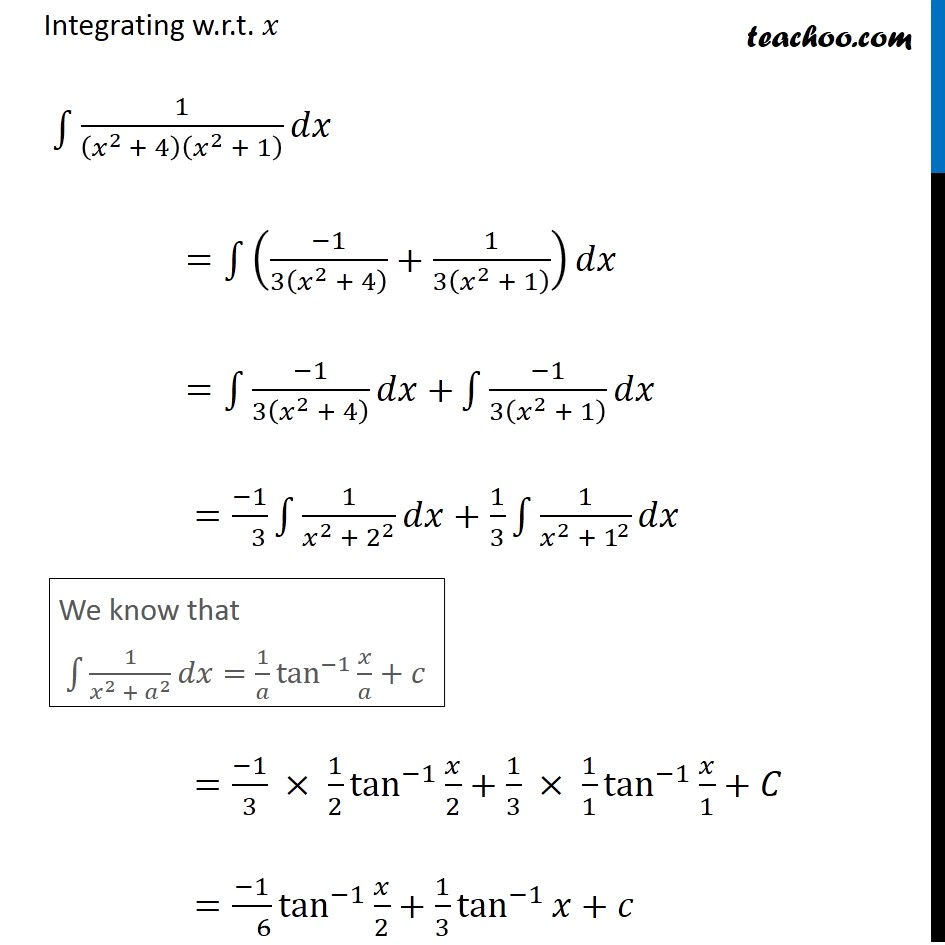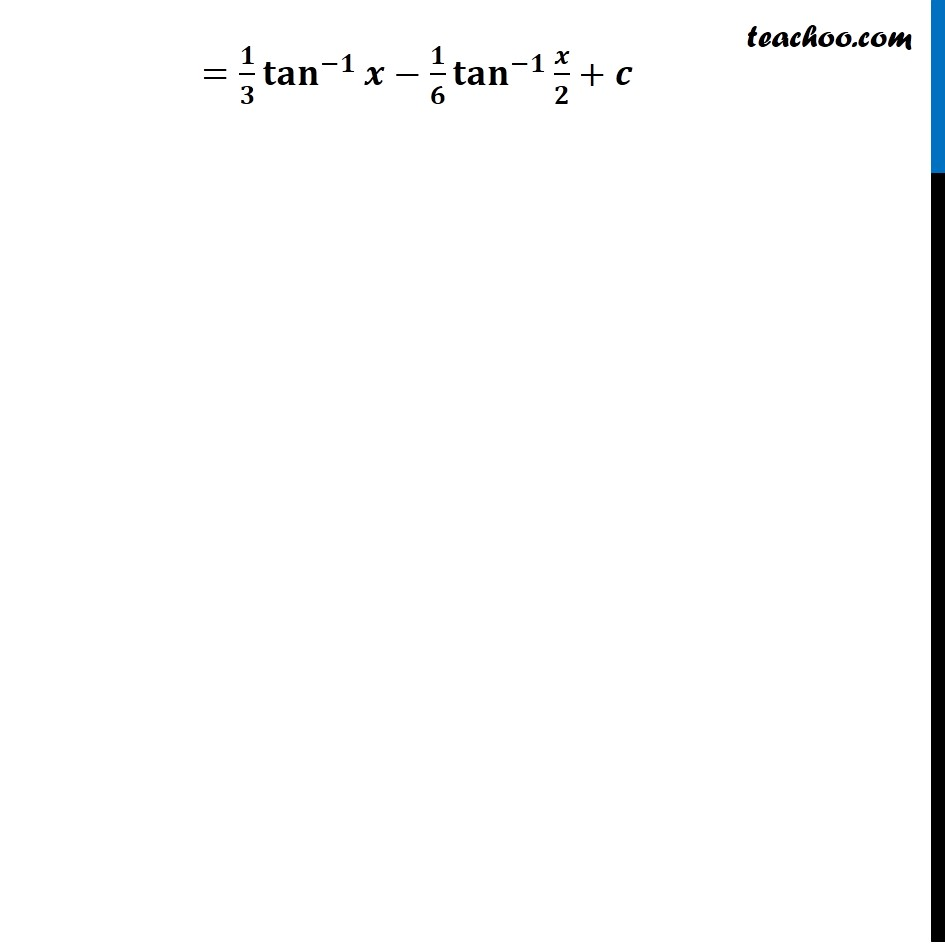Introducing your new favourite teacher - Teachoo Black, at only ₹83 per month

### Transcript

Misc 14 Integrate the function 1/((𝑥^2 + 1)(𝑥^2 + 4) ) Integrating 1/(𝑥^2 + 1)(𝑥^2 + 4) Let 𝑥^2=𝑦 1/(𝑥^2 + 1)(𝑥^2 + 4) =1/(𝑦 + 4)(𝑦 + 1) Hence we can write 1/(𝑦 + 4)(𝑦 + 1) =𝐴/((𝑦 + 4) )+𝐵/((𝑦 + 1) ) 1/(𝑦 + 4)(𝑦 + 1) =(𝐴(𝑦 + 1)+𝐵(𝑦 + 4))/(𝑦 + 4)(𝑦 + 1) Canceling denominators 1 = A(𝑦+1)+B(𝑦+4) Putting 𝒚=−𝟏 1= A (−1+1)+B(−1+4) 1= A ×0+ B (3) 1=3B B =1/3 Putting 𝒚=−𝟒 1= A(−4+1)+ B(−4+4) 1= A(−3)+ B(0) 1=−3A A =(−1)/3 Therefore we can write 1/(𝑦 + 4)(𝑦 + 1) =𝐴/((𝑦 + 4) )+𝐵/((𝑦 + 1) ) 1/(𝑦 + 4)(𝑦 + 1) =(((−1)/( 3)))/((𝑦 + 4) )+((1/3))/((𝑦 + 1) ) Putting back 𝑦=𝑥^2 1/(𝑥^2 + 4)(𝑥^2 + 1) =(((−1)/( 3)))/((𝑥^2 + 4) )+((( 1)/( 3)))/((𝑥^2+ 1) ) = (−1)/3(𝑥^2 + 4) +1/3(𝑥^(2 )+ 1) Integrating w.r.t. 𝑥 ∫1▒█(1/(𝑥^(2 )+ 4)(𝑥^2 + 1) 𝑑𝑥) =∫1▒((−1)/3(𝑥^2 + 4) +1/3(𝑥^2 + 1) )𝑑𝑥 =∫1▒〖(−1)/3(𝑥^2 + 4) 𝑑𝑥+〗 ∫1▒〖(−1)/3(𝑥^2 + 1) 𝑑𝑥〗 =(−1)/( 3) ∫1▒〖1/(𝑥^2 + 2^2 ) 𝑑𝑥+1/3 ∫1▒〖1/(𝑥^2 + 1^2 ) 𝑑𝑥〗〗 =(−1)/3 × 1/2 tan^(−1)⁡〖𝑥/2+1/3 × 1/1 tan^(−1)⁡〖𝑥/1+𝐶〗 〗 =(−1)/( 6) tan^(−1)⁡〖𝑥/2+1/3 tan^(−1)⁡〖𝑥+𝑐〗 〗 We know that ∫1▒〖1/(𝑥^2 + 𝑎^2 ) 𝑑𝑥=1/𝑎 tan^(−1)⁡〖𝑥/𝑎+𝑐〗 〗 =𝟏/𝟑 〖𝐭𝐚𝐧〗^(−𝟏)⁡〖𝒙−𝟏/𝟔 〖𝐭𝐚𝐧〗^(−𝟏)⁡〖𝒙/𝟐+𝒄〗 〗